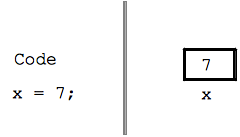# Variables and =

This is a short section, to add the idea of variables to the code.

• A "variable" is like a box that holds a value
• `x = 7;`
• The above line stores the value 7 into the variable named x
• Later appearances of x in the code retrieve the value from the box
• Using = in this way is called "variable assignment"x = 7; print(x); print("lucky", x); print("x is", x);

• Experiments: try assigning (=) these values to x: 8, "hi"
• The name x can be anything we choose, so long as we are consistent
--Try changing it to xyz throughout
• The Point: store a value once, use it on several lines, can save repetition
• Not the same as = in algebra, that means two things are equal forever
• = in code is simple -- just put a value in a box when that line runs

Variables work as a shorthand -- we = assign a value into a variable, and then use that variable on later lines to retrieve that value. In the simplest case, this just works to avoid repeating a value: we store the value once, and then can use it many times. All computer languages have some form of variable like this -- storing and retrieving values.

## Code Example - The Curse

Change the code below so it produces the following output. Use a variable to store the string "Alice" in a variable on the first line like `x = "Alice";`, then use the variable x on the later lines. In this way, changing just the first line to use the value "Bob" or "Zoe" or whatever changes the output of the whole program.

```Alice Alice Alice Alice
In high school I had a crush on Alice
Now the Alice curse is lifted
```

 print(1, 2, "hi");